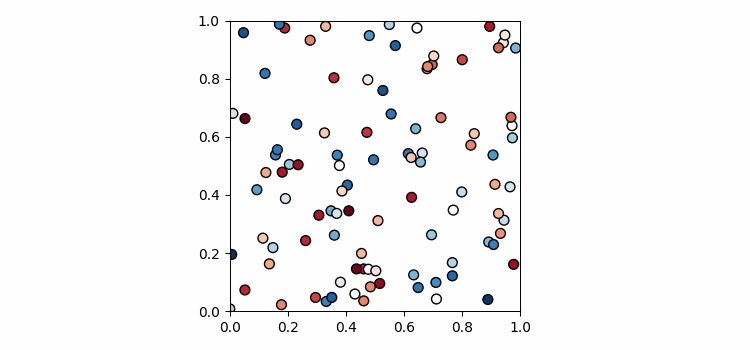# Saving scatterplot animations with matplotlib

To save scatterplot animations with matplotlib, we can take the following steps −

• Set the figure size and adjust the padding between and around the subplots.
• Initialize four variables, steps, nodes, positions and solutions.
• Append positions and solutions values in the list.
• Create a figure and a set of subplots.
• Initialize a variable for marker size.
• Configure the grid lines.
• Make an animation by repeatedly calling a function *animate*, to clear the axis, add new axis sublot, and plot scatter points on the axis.
• Save the animated scatter plot as a .gif file.

## Example

import matplotlib.pyplot as plt
import matplotlib.animation as animation
import numpy as np

plt.rcParams["figure.figsize"] = [7.50, 3.50]
plt.rcParams["figure.autolayout"] = True

steps = 50
nodes = 100
positions = []
solutions = []

for i in range(steps):
positions.append(np.random.rand(2, nodes))
solutions.append(np.random.random(nodes))

fig, ax = plt.subplots()
marker_size = 50

def animate(i):
fig.clear()
ax = fig.add_subplot(111, aspect='equal', autoscale_on=False, xlim=(0, 1), ylim=(0, 1))
ax.set_xlim(0, 1)
ax.set_ylim(0, 1)
s = ax.scatter(positions[i], positions[i], s=marker_size, c=solutions[i], cmap="RdBu_r", marker="o", edgecolor='black')

plt.grid(b=None)
ani = animation.FuncAnimation(fig, animate, interval=100, frames=range(steps))

ani.save('animation.gif', writer='pillow')

## Output